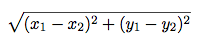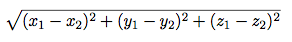## Instructions

Download hw01.zip. Inside the archive, you will find starter files for the questions in this homework, along with a copy of the OK autograder.

Submission: When you are done, submit with `python3 ok --submit`. You may submit more than once before the deadline; only the final submission will be scored. Check that you have successfully submitted your code on okpy.org.

Readings: This homework relies on following references:

To submit: run `ok` with the `--submit` option:

``python3 ok --submit``

## Review from lab

### Division

Let's compare the different division-related operators in Python:

True Division
(decimal division)
The `/` Operator
Floor Division
(integer division)
The `//` Operator
Modulo
(similar to a remainder)
The `%` Operator
``````>>> 1 / 5
0.2

>>> 25 / 4
6.25

>>> 5 / 0
ZeroDivisionError
``````
``````>>> 1 // 5
0

>>> 25 // 4
6

>>> 5 // 0
ZeroDivisionError
``````
``````>>> 1 % 5
1

>>> 25 % 4
1

>>> 5 % 0
ZeroDivisionError
``````

Note that floor division and modulo both return an integer while true division always returns a floating point number.
For now, the only difference you need to understand between integers and floats is that integers are positive or negative whole numbers, while floats are positive or negative decimals.

One useful technique involving the `%` operator is to check whether a number `x` is divisible by another number `y`:

``x % y == 0``

For example, in order to check if `x` is an even number:

``x % 2 == 0``

### Floats and precedence

Floating point numbers (floats) behave a lot like real numbers. You can identify a float by the decimal point. All floats have decimal points. To write a floating point number (as a literal) you must add a decimal point!

``````>>> 3.141592
3.141592
>>> 2*3.141592 # you can mix ints and floats
6.283184
>>> pie = 3.141592 # you can assign values to variables
>>> pie
3.141592
>>> pie/pie
1.0
>>> pie/pie == 1 # a float can be equal in value to an int
True
>>> from math import pi   # here is a better pi
>>> pi
3.141592653589793
>>> 5.0/3.0  # this is division of floats, not ints
1.6666666666666667
>>> 2**(1/2) # square root - isn't that transcendental?
1.4142135623730951``````

Expressions follow operator precedence (just like in math). Operations are performed one at a time in a specific order. Parenthesis are used to specify order (again, just like in math - remember PEMDAS?).

``````>>> 2 + 3 - 4 + 5   # equal precedence, left to right
6
>>> 2 + 3 - (4 + 5) # order matters - parentheses are your friend
-4
>>> (((2 + 3) - 4) + 5) # explicit order of the first example
6
>>> 2 + 3 * 4   # * and / bind more tightly than + or -
14
>>> 2 + (3 * 4)
14
>>> (2 + 3) * 4
20
>>> 2 + 3 / 4 * 5  # what about * and / ?
5.75``````

An expression can have multiple return values, called a tuple:

``````>>> 2, 3
(2, 3)
>>> x, y = 1, pi
>>> y
3.141592653589793``````

### Strings

It is very useful to be able to write programs that operate on strings, not just numbers. Without strings, web browsers and word processors would be like the matrix! Just as with ints, floats, and booleans, strings are a data type and have certain operators defined on them:

``````>>> 'cal'           # a string literal is a sequence of characters in quotes
'cal'
>>> "rocks"         # either kind of quote, but they need to match
'rocks'
>>> "cal" + "rocks"   # + is concatenation
'calrocks'
>>> 'cal' * 3         # * is replication
'calcalcal'
>>> 'cal' == "cal"  # equality is if they are the same string (notice the single and double quotes)
True
>>> 'Cal' == 'cal'  # case sensitive
False
>>> 'Cal' < 'cal'   # lexicographic ordering, with upper before lower case
True
>>> 'you' is not 'me'
True``````

## Problems

### Question 1: Oddly we go

Define `odd`, which takes an integer and returns whether it is odd. Your solution will look like `return <expression>`.

`````` def odd(number):
"""Return whether the number is odd.

>>> odd(2)
False
>>> odd(5)
True
"""

Use OK to test your code:

``python3 ok -q odd``

### Question 2: Distance

Implement a function called `distance(x1, y1, x2, y2)` where:

• `x1` and `y1` form an x-y coordinate pair
• `x2` and `y2` form an x-y coordinate pair

`distance` returns the Euclidean distance between the two points. Use the following formula:``````from math import sqrt

def distance(x1, y1, x2, y2):
"""Calculates the Euclidian distance between two points (x1, y1) and (x2, y2)

>>> distance(1, 1, 1, 2)
1.0
>>> distance(1, 3, 1, 1)
2.0
>>> distance(1, 2, 3, 4)
2.8284271247461903
"""

Use OK to test your code:

``python3 ok -q distance``

### Question 3: Distance (3-D)

Now, let us edit this program to get the distance between two 3-dimensional coordinates. Your `distance3d` function should take six arguments and compute the following:``````def distance3d(x1, y1, z1, x2, y2, z2):
"""Calculates the 3D Euclidian distance between two points (x1, y1, z1) and
(x2, y2, z2).

>>> distance3d(1, 1, 1, 1, 2, 1)
1.0
>>> distance3d(2, 3, 5, 5, 8, 3)
6.164414002968976
"""

Use OK to test your code:

``python3 ok -q distance3d``

We've seen that we can name a value by assigning it to a variable. Functions are objects too. Try typing the name of a function you have defined or imported into the python interpreter. Try importing a function as in below.
Try assigning it, e.g., `>>> funfun = add`. Try calling this. What is its type?

Fill in the blanks in the following function definition for adding `a` to the absolute value of `b`, without calling `abs` or defining any new functions.

Hint: Look at the top line.

``````from operator import add, sub

def a_plus_abs_b(a, b):
"""Return a+abs(b), but without calling abs.

>>> a_plus_abs_b(2, 3)
5
>>> a_plus_abs_b(2, -3)
5
>>> a_plus_abs_b(-5, -1)
-4
"""
if b < 0:
f = _____
else:
f = _____
return f(a, b) # You can replace this line, but don't have to.``````

Use OK to test your code:

``python3 ok -q a_plus_abs_b``

Complete the function so that it returns a tuple that contains both roots of a quadratic polynomial using the quadratic formula. The first element in the tuple should be the "minus" case and the second element should be the "plus" case. Your solution must call the `sqrt` function exactly once.

See here for a basic tuple example.

``````from math import sqrt

"""
(-1.0, 1.0)
(-1.0, -1.0)
(-4.0, 1.0)
"""

Use OK to test your code:

``python3 ok -q quadratic``

### Question 6: Falling Factorial

Let's write a function `falling`, which is a "falling" factorial that takes two arguments, `n` and `k`, and returns the product of `k` consecutive numbers, starting from `n` and working downwards.

If `k` is larger than n, only multiply up to n consecutive numbers!

``````def falling(n, k):
"""Compute the falling factorial of n to depth k.

>>> falling(6, 3)  # 6 * 5 * 4
120
>>> falling(4, 3)  # 4 * 3 * 2
24
>>> falling(4, 1)  # 4
4
>>> falling(4, 0)
1
"""

Use OK to test your code:

``python3 ok -q falling``

### Question 7: Hailstone

Complete this question using iteration!

Douglas Hofstadter's Pulitzer-prize-winning book, Gödel, Escher, Bach, poses the following mathematical puzzle:

1. Pick a positive integer `n` as the start.
2. If `n` is even, divide it by 2.
3. If `n` is odd, multiply it by 3 and add 1.
4. Continue this process until `n` is 1.

The sequence of values of `n` is often called a Hailstone sequence, because hailstones also travel up and down in the atmosphere before falling to earth. Write a function that takes a single argument with formal parameter name `n`, prints out the hailstone sequence starting at `n`, and returns the number of steps in the sequence:

``````def hailstone(n):
"""Print the hailstone sequence starting at n and return its
length.

>>> a = hailstone(10)
10
5
16
8
4
2
1
>>> a
7
"""

Hailstone sequences can get quite long! Try 27. What's the longest you can find?

Use OK to test your code:

``python3 ok -q hailstone``

## Submit

Make sure to submit this assignment by running:

``python3 ok --submit``

## Optional Questions

### Question 8: Harmonic Mean

Implement `harmonic_mean`, which returns the harmonic mean of two positive numbers `x` and `y`. The harmonic mean of 2 numbers is 2 divided by the sum of the reciprocals of the numbers. (The reciprocal of `x` is `1/x`.)

``````def harmonic_mean(x, y):
"""Return the harmonic mean of x and y.

>>> harmonic_mean(2, 6)
3.0
>>> harmonic_mean(1, 1)
1.0
>>> harmonic_mean(2.5, 7.5)
3.75
>>> harmonic_mean(4, 12)
6.0
"""

Use OK to test your code:

``python3 ok -q harmonic_mean``

### Question 9: Two of three

Write a function that takes three positive numbers and returns the sum of the squares of the two largest numbers. Use only a single expression for the body of the function.

``````def two_of_three(a, b, c):
"""Return x*x + y*y, where x and y are the two largest members of the
positive numbers a, b, and c.

>>> two_of_three(1, 2, 3)
13
>>> two_of_three(5, 3, 1)
34
>>> two_of_three(10, 2, 8)
164
>>> two_of_three(5, 5, 5)
50
"""
``python3 ok -q two_of_three``